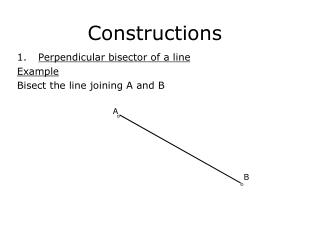DownloadDownload PresentationConstructions

# Constructions

Download Presentation## Constructions

- - - - - - - - - - - - - - - - - - - - - - - - - - - E N D - - - - - - - - - - - - - - - - - - - - - - - - - - -
##### Presentation Transcript

1. Constructions A Perpendicular bisector of a line Example Bisect the line joining A and B B

2. Example Bisect the line PQ Q P The line drawn is also known as a locus of all points which are equidistant (equal in distance) from P and Q.

3. 2. Bisecting an angle Example Bisect the angle given by A A

4. Example Bisect the angle given by ABC C A B The line drawn is also known as a locus of all points which are equidistant (equal in distance) from AB and BC

5. 3. Dropping a perpendicular from a point to a line Example Drop a perpendicular from the point P to the line AB P A B

6. Example Drop a perpendicular from the point P to the line LM L P M